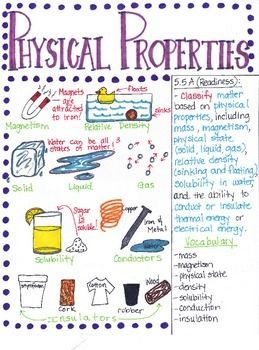Physical Properties of Matter
4 years ago
gatlinba
Save
Edit
Host a game
Live GameLive
Homework
Solo Practice
Practice15 QuestionsShow answers
• Question 1
30 secondsQ. What is the volume of the rock? The water level is displaced from 20 mL to 23 mL with the rock.
3 mL
23 mL
20 mL
43 mL
• Question 2
30 seconds
Q. In which state does matter completely fill its closed container?
Gas
Liquid
Solid
Ice
• Question 3
30 seconds
Q. Which state of matter has a fixed shape and volume and it's molecules move very slowly?
Liquid
Gas
Solid
Plasma
• Question 4
30 seconds
Q. Anything that has mass and takes up space is called
volume
volume
matter
magnetism
electricity
• Question 5
30 seconds
Q. How we describe and classify matter is called
properties
matter
mass
volume
• Question 6
30 seconds
Q. What are the three states of matter?
gas, liquid, mass
solid, liquid, gas
mass, volume, thermal energy
gas, temperature, solids
• Question 7
30 seconds
Q. Which physical property is the amount of space something takes up and can be measured with a graduated cylinder?
conductivity
relative density
thermal energy
volume
• Question 8
30 seconds
Q. Each of these objects is an example of what?house, desk, pencil, car
metal
wood
plastic
matter
• Question 9
30 seconds
Q. How did this student classify these objects?Balloon, orange, ball
Weight
color
texture
shape
• Question 10
60 secondsQ. Which of these best describes the texture of this metal pan?
small
smooth
flexible
shiny
• Question 11
30 seconds
Q. When paper is folded to make an origami bookmark, what physical property of the paper changes?
mass
weight
shape
smell
• Question 12
120 seconds
Q. Which tool would be used to measure the mass of an object?
balance
meter stick
spring scale
• Question 13
30 seconds
Q. Example for Physical Property
Taste
explodes
• Question 14
30 seconds
Q. Example for Physical property